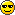# supercritical fluid

A supercritical fluid is any substance at a temperature and pressure above its critical point. It can effuse through solids like a gas, and dissolve materials like a liquid. In addition, close to the critical point, small changes in pressure or temperature result in large changes in density, allowing many properties of a supercritical fluid to be "fine-tuned". Supercritical fluids are suitable as a substitute for organic solvents in a range of industrial and laboratory processes. Carbon dioxide and water are the most commonly used supercritical fluids, being used for decaffeination and power generation, respectively.

In addition, there is no surface tension in a supercritical fluid, as there is no liquid/gas phase boundary. By changing the pressure and temperature of the fluid, the properties can be â€œtunedâ€ to be more liquid- or more gas-like. One of the most important properties is the solubility of material in the fluid. Solubility in a supercritical fluid tends to increase with density of the fluid (at constant temperature). Since density increases with pressure, solubility tends to increase with pressure. The relationship with temperature is a little more complicated. At constant density, solubility will increase with temperature. However, close to the critical point, the density can drop sharply with a slight increase in temperature. Therefore, close to the critical temperature, solubility often drops with increasing temperature, then rises again.

All supercritical fluids are completely miscible with each other so for a mixture a single phase can be guaranteed if the critical point of the mixture is exceeded. The critical point of a binary mixture can be estimated as the arithmetic mean of the critical temperatures and pressures of the two components,

Tc(mix) = (mole fraction A) x TcA + (mole fractionx TcB.

For greater accuracy, the critical point can be calculated using equations of state, such as the Peng Robinson, or group contribution methods. Other properties, such as density, can also be calculated using equations of state. (wikipedia)

Handy Calculator for density, enthalpy, entropy and other thermodynamic data of supercritical Carbon Dioxide
Animated presentation describing what a supercritical fluid is

Created by Dale Pond. Last Modification: Monday February 14, 2011 02:34:02 MST by Dale Pond.